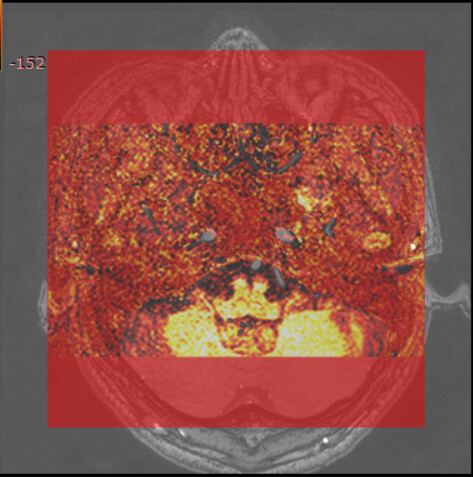# How to understand the Image Position and vtkImageData.GetOrigin()?

It’s difficult for me to understand the Image Position (0018, 5100) and the vtkImageData.GetOrigin(). Then, I show it as following:

``````import vtk, time
from vedo import Point

img.SetFileName('C:\\Users\\MLoong\\Desktop\\dicom_data\\Chang Cheng\\TOF\\IM_0198')
img.Update()

origin = img.GetOutput().GetOrigin()
print('origin1: ', origin)
pOrigin = Point(pos=origin, c='red') # the color of origin point is red

position = [-108.38450836769, -165.12128686904, 97.8158312461331] # I don't know how to obtain patient position from vtk reader
# thus, I give the position from RadiAnt
pPosition = Point(pos=position, c='green')  # the color of position point is green

imageActor = vtk.vtkImageActor()
windowLevel = vtk.vtkImageMapToWindowLevelColors()
imageActor.GetMapper().SetInputConnection(windowLevel.GetOutputPort())

ren = vtk.vtkRenderer()
ren.SetBackground(0.1, 0.2, 0.4)

renWin = vtk.vtkRenderWindow()
renWin.SetSize(400, 400)

iren = vtk.vtkRenderWindowInteractor()
iren.SetRenderWindow(renWin)

start = time.time()

windowLevel.SetInputData(img.GetOutput())
windowLevel.Update()

renWin.Render()

iren.Start()
``````

And the result is:

In the above figure, the red point is the origin point, and the green point is the image position point. From this figure, my understanding is that the vtkImageData.GetOrigin() point is the bottom left point of image, and the image position point is a point from the scanner.

Moreover, I have two image (from RadiAnt) as following:

Then, I print both vtkImageData.GetOrigin() of them, and the result are both `(0, 0, 0)`, then I use `vtkImageBlend` to show them:

From this figure, we can see that the bottom left point is the same for the two images, which demonstrate that the vtkImageData.GetOrigin() of `(0, 0, 0)` is right. However, in the last RadiAnt figure, we can see the artery (red arrow) is the same, but it is not in this blended figure.

Moreover, the RadiAnt provide a `Fusion` function, and the result is:This RadiAnt fusion figure is different from the vtkImageBlend result.

Does all vtkImageData.GetOrigin() return (0, 0, 0)??

If your goal is to learn more about medical imaging: These questions are all solved in all existing medical image viewers. You can find the answers by reviewing source code of 3D Slicer, MITK, ITK-snap, etc.

If your goal is to implement a clinical application: Do not start from scratch, ignoring decades of work in this field. Instead build on an existing application platform, such as 3D Slicer or MITK.